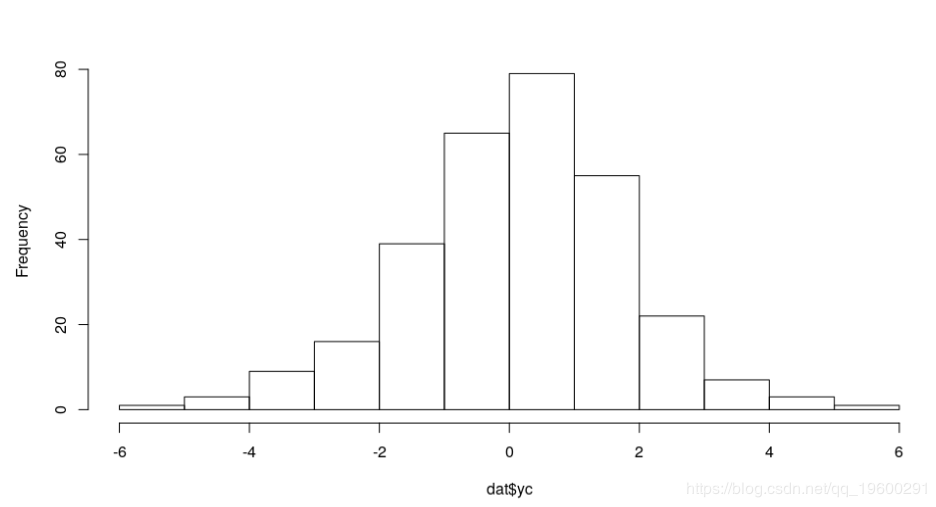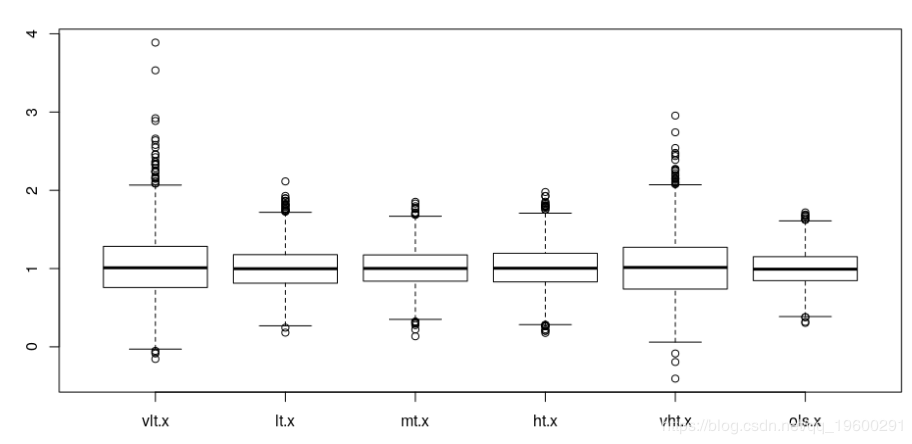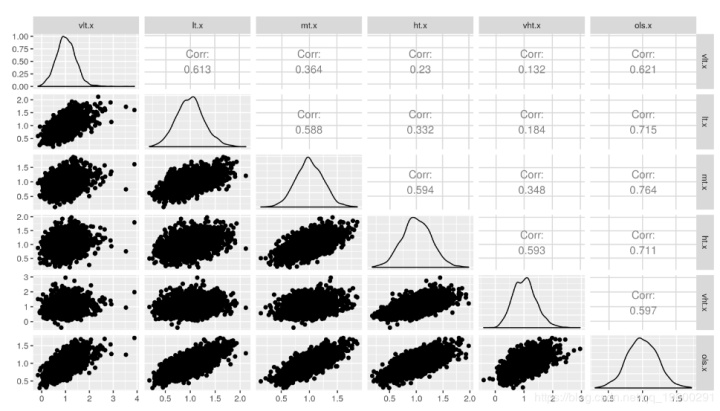• R语言连续变量等级划分 ###################等级划分################### ###数据读取 dat <- read.csv("F:/Desktop/ccv.csv") # ##查看数据结构 # str(dat) ##########以按HT(优势树高)划分等级为例######...
R语言连续变量等级划分
###################等级划分###################
###数据读取
# ##查看数据结构
#  str(dat)

##########以按HT(优势树高)划分等级为例##################

###查看HT最小值、最大值以及均值
a <- min(dat$HT) b <- max(dat$HT)
c <- mean(dat$HT) print(list(min = a, max = b, mean = c)) ###按5m一个等级划分 # ##method1 # #从0开始向最大值划分的等级（水平）数：num；ceiling函数表示向上取整 # num <- ceiling(b/5) # # # #最接近HT最大值的能被“划分距离（这里HT按5m划分）”整除的数：n; 这里HT最大值为b = 22.3, 那么n = 5*5=25 # n <- num*5 ##method2 #不从0开始划分的等级数 num <- ceiling((b-a)/5)+1 #不从0开始划分 n1 <- floor(a/5)*5 n2 <- ceiling(b/5)*5 ##采用cut函数将数据分组 # breaks <- seq(0,n,by = 5)#(method1) breaks <- seq(n1,n2,by = 5)#(method2) cluster_HT_5 <- cut(dat$HT,breaks = breaks)

##各水平按默认排序重命名
levels(cluster_HT_5) <- c(1:num)

##确定各等级（水平）样本量
plot(cluster_HT_5)
table(cluster_HT_5)

##保存等级划分结果
x1 <- cbind(dat,cluster_HT_5)

write.csv(x1,file = "F:/Desktop/cluster_HT_5_method2.csv")

展开全文• 原文链接：R语言是否对二分连续变量执行逻辑回归​tecdat.cn教育或医学的标准情况是我们有一个持续的衡量标准，但随后我们对那些具有临床/实际意义的连续措施有了切入点。一个例子是BMI。您可以通过70分作为成绩测试...

原文链接：

R语言是否对二分连续变量执行逻辑回归​tecdat.cn教育或医学的标准情况是我们有一个持续的衡量标准，但随后我们对那些具有临床/实际意义的连续措施有了切入点。一个例子是BMI。您可以通过70分作为成绩测试进行成绩测试。当这种情况发生时，研究人员有时可能会对BMI模型超过30或通过/失败感兴趣。实质性问题通常属于模拟某人超过/低于该临床显着阈值的概率的线条。因此，我们使用逻辑回归等方法对连续测量进行二分，并分析新的二元变量。
那么这种方法在实践中如何运作？任何尝试在使用逻辑回归进行分析之前在不同阈值下对连续变量进行二分法的人都会知道估计的系数会发生变化并且可能会发生很大变化,这是否与声称结果不应取决于阈值一致？我们可以使用模拟。首先，我将介绍数据生成过程：

dat <- data.frame(x = rbinom(300, 1, .5))
# Outcome ys = intercept of -0.5, the coefficient of x is 1 and there is logistic error
dat$yc <- -.5 + dat$x + rlogis(nrow(dat))


hist(dat$yc, main = "")​ 然后，我们可以yc在不同点上对结果进行二分，以确定这是否会影响x我们使用逻辑回归的估计系数： coef(glm((yc > -2) ~ x, binomial, dat))["x"] # Cut it at extreme -2 x 0.9619012 coef(glm((yc > 0) ~ x, binomial, dat))["x"] # Cut it at midpoint 0 x 1.002632 coef(glm((yc > 2) ~ x, binomial, dat))["x"] # Cut it at extreme 2 x 0.8382662  如果我们yc直接应用线性回归怎么办？ # First, we create an equation to extract the coefficients and # transform them using the transform to logit formula above. trans <- function (fit, scale = pi / sqrt(3)) { scale * coef(fit) / sigma(fit) } trans(lm(yc ~ x, dat))["x"] x 1.157362  所有这些数字彼此并没有太大的不同。现在我们可以多次重复此过程来比较结果中的模式。我会重复2500次： colMeans(res <- t(replicate(2500, { dat <- data.frame(x = rbinom(300, 1, .5)) dat$yc <- -.5 + dat\$x + rlogis(nrow(dat))

# v for very; l/m/h for low/middle/high; and t for threshold; ols for regular regression
c(vlt = coef(glm((yc > -2) ~ x, binomial, dat))["x"],
lt = coef(glm((yc > -1) ~ x, binomial, dat))["x"],
mt = coef(glm((yc > 0) ~ x, binomial, dat))["x"],
ht = coef(glm((yc > 1) ~ x, binomial, dat))["x"],
vht = coef(glm((yc > 2) ~ x, binomial, dat))["x"],
ols = trans(lm(yc ~ x, dat))["x"])
})))
vlt.x      lt.x      mt.x      ht.x     vht.x     ols.x
1.0252116 1.0020822 1.0049156 1.0101613 1.0267511 0.9983772


这些数字是不同方法的平均回归系数。
boxplot(res)​
我们看到虽然平均值大致相同，但当阈值极端时，估计的系数变化更大。最小变量系数是变换后的线性回归系数，因此当我们使用线性回归方法时，结果稳定。
不同方法之间的估计系数模式如何？

 ggpairs(as.data.frame(res))​
我们看到当阈值非常低时估计系数与阈值非常高时的估计系数非常弱相关（.13）。这些差异只是反映了阈值，在实际数据分析中可能会产生误导。

基于这些结果，预测因子与结果之间的关系也可能因结果的不同分位数而不同--分位数回归探讨的情况。可以使用分位数回归方法来查看原始连续结果中是否存在这种情况。
非常感谢您阅读本文，有任何问题请在下面留言！

展开全文• I am working on data collection by R on Win7.The given data is:var1 var2 valueI need to do grouping by var1 and then for each var1 , do grouping by var2.Then, the output is column vectors of val...I am working on data collection by R on Win7.
The given data is:
var1 var2 value
I need to do grouping by var1 and then for each var1 , do grouping by var2.
Then, the output is column vectors of values that are associated with the same var1 and var2. Here, var1 and var2 are like keys.
Example,
var1 var2 value
1 56 649578
2 17 357835
1 88 572397
2 90 357289
1 56 427352
2 17 498455
1 88 354623
2 90 678658
The result should be
var1 var2 value
1 56 649578
1 56 427352
1 88 354623
1 88 572397
2 17 357835
2 17 498455
2 90 357289
2 90 678658
And, I need to print the values in a CSV file as
For var 1 as 1:
649578 354623
427352 572397
For var 1 as 2:
357835 357289
498455 678658
And, I also need to print the values in a CSV file as
For var 1 = 1:
1 56 649578
1 56 427352
1 88 354623
1 88 572397
For var1 = 2:
2 17 357835
2 17 498455
2 90 357289
2 90 678658
How to do it ?
I found some posts, which are not directly useful.
Update:
How to choose and print the values that are associated with each unique var2 ?
Are there dictionary data structure in R?
解决方案
This is relatively close to what you are looking for I believe, but not quite the same. It should provide some help though
library(reshape2)
library(plyr)
dat
dat
dat
dat.new.new
the second dat call using order() will order the results as you requested, and the dat.new.new data frame is close to what you were looking for.
bonus points for catching the KidCudi reference

展开全文• 按指定行进行分组计数 按指定字段进行分组：计数为一个字段下分几类：用长变宽函数pivot_wider（） 按照制定顺序进行行排序：用一个带顺序的表控制合并表的关键字full_join（） 按照制定顺序进行列排序：SELECT...

导入相关包导入文件按指定行进行分组计数按指定字段进行分组：计数为一个字段下分几类：用长变宽函数pivot_wider（）按照制定顺序进行行排序：用一个带顺序的表控制合并表的关键字full_join（）按照制定顺序进行列排序：SELECT（）导出文件 library(tidyverse)
wp0 <- read_excel("wp.xlsx", sheet = "wp0")
wp1 <- read_excel("wp.xlsx", sheet = "wp1")

#-----------------------------板块------------------------------------
wp0 %>% count()
df0 <-wp0 %>% count(板块名称)
names(df0)[names(df0) == 'n'] <- 'n0'

wp1 %>% count()
df1 <-wp1 %>% count(板块名称)
df2 <-wp1 %>% count(板块名称,租赁来源) %>% pivot_wider( names_from = "租赁来源", values_from = "n")
df3 <-wp1 %>% count(板块名称,租赁方式)%>% pivot_wider( names_from = "租赁方式", values_from = "n")
df4 <-wp1 %>% count(板块名称,建筑面积)%>% pivot_wider( names_from = "建筑面积", values_from = "n")
df5 <-wp1 %>% count(板块名称,租金水平)%>% pivot_wider( names_from = "租金水平", values_from = "n")
df6 <- wp1 %>% group_by(板块名称) %>% summarize(租金单价=mean(租金单价))
#
x <- c('广埠屯板块','洪山东板块','洪山西板块','狮子山洪山板块','张家湾板块','和平东板块','和平西板块','青菱板块','关山东板块','光谷广场板块','软件园板块','关山西板块')
y <- c(1,2,3,4,5,6,7,8,9,10,11,12)
df_num <- data.frame(板块名称= x, num = y )

wp_bankuai <- df_num  %>% full_join(df0)%>% full_join(df1)%>% full_join(df2)%>% full_join(df3)%>% full_join(df4)%>% full_join(df5)%>% full_join(df6)
###！！！列排序谨慎用，由于相关标度无数据需要手动确认是否删除
'''
wp_bankuai <-select(wp_bankuai,
板块名称,num,n0,
中介,长租公寓,中介,
整租,分租,
≤20㎡,（20,60]㎡,（60,90]㎡,（90,140]㎡,140㎡以上,
≤500元,（500,1000]元,（1000,2000]元,（2000,5000]元,＞5000元,
租金单价
)
'''
# 导出文件
write_csv(wp_bankuai, "wp_bankuai.csv")
#-----------------------------街道------------------------------------
wp0 %>% count()
df0 <-wp0 %>% count(街道名称)
names(df0)[names(df0) == 'n'] <- 'n0'

wp1 %>% count()
df1 <-wp1 %>% count(街道名称)
df2 <-wp1 %>% count(街道名称,租赁来源) %>% pivot_wider( names_from = "租赁来源", values_from = "n")
df3 <-wp1 %>% count(街道名称,租赁方式)%>% pivot_wider( names_from = "租赁方式", values_from = "n")
df4 <-wp1 %>% count(街道名称,建筑面积)%>% pivot_wider( names_from = "建筑面积", values_from = "n")
df5 <-wp1 %>% count(街道名称,租金水平)%>% pivot_wider( names_from = "租金水平", values_from = "n")
df6 <- wp1 %>% group_by(街道名称) %>% summarize(租金单价=mean(租金单价))
#
x <- c('珞南街道','洪山街道','关山街道','卓刀泉街道','狮子山街道','梨园街道','和平街道','张家湾街道','青菱街道')
y <- c(1,2,3,4,5,6,7,8,9)
df_num <- data.frame(街道名称= x, num = y )

wp_jiedao <- df_num  %>% full_join(df0)%>% full_join(df1)%>% full_join(df2)%>% full_join(df3)%>% full_join(df4)%>% full_join(df5)%>% full_join(df6)
''
wp_jiedao <-select(wp_jiedao,
板块名称,num,n0,
中介,长租公寓,中介,
整租,分租,
≤20㎡,（20,60]㎡,（60,90]㎡,（90,140]㎡,140㎡以上,
≤500元,（500,1000]元,（1000,2000]元,（2000,5000]元,＞5000元,
租金单价
)
''
# 导出文件
write_csv(wp_jiedao, "wp_jiedao.csv")  
展开全文• 此后还要进一步探索变量分组两两之间的均值关系，即事后检验 (post-hoc test)。 基于不同的对第I类错误的控制方法，有多种事后检验的方法可以选择。 TukeyHSD检验：TukeyHSD() 语法：TukeyHSD(aov模型名) > TukeyHSD...统计学
• 论文中常见的分组箱型图和分组条形图可以直观的比较方法的效果，以一个图显示多个方法在多个数据集上的AUC或AUPR，包含2个分类变量和1个连续变量分组条形图 数据 分组箱型图 ...ggplot2
• 数据离散化处理属于数据预处理的一个过程，R语言在数据处理上有天然的优势，也有直接用于离散化计算的包，无监督的离散化可以用infotheo包，有监督的离散化可以用discretization包来处理复杂的离散化操作。...
• 社会科学研究经常会遇到“超多变量”的情况——多量表、多维度、多题项，以及复杂的正反计分题……如何更高效地计算...今天我们就来掌握如何使用R语言优雅地计算超多变量（包括计算总分、计算平均值、缺失值处理、数...
• R语言简介: R语言是一门专用于统计分析的语言,有大量的内置函数和第三方库来制作基于数据的表格 准备工作 安装R语言 https://cran.rstudio.com/bin/windows/base/R-3.4.3-win.exe 安装Rstudio ...
• 生存分析之R包survival的单变量和多变量Cox回归续前文生存分析(Survival Analysis)。在前文初步简介了生存分析的概念，以及展示了一种生存分析模型Kaplan-Meier的使用。Kaplan-Meier是一种非参数的单变量分析方法，...
• R语言︱数据集分组大型数据集通常是高度结构化的，结构使得我们可以按不同的方式分组，有时候我们需要关注单个组的数据片断，有时需要聚合不同组内的信息，并相互比较。一、日期分组1、关于时间的包都有很多很好的...data.table 排序
• 数据可视化_R语言多种方式呈现分组数据（箱形图、小提琴图、散点图、误差条图等）准备分组的离散变量分组连续变量箱形图(Box plots)小提琴图(Violin plots)点图(Dot plots)一维散点图(Stripcharts)Sinaplot带有...数据可视化 ggplot2
• 对于无序分类变量的分析，应先按类别分组，清点各组的观察单位数，编制分类变量的频数表，所得资料为无序分类资料，亦称计数资料。  有序分类变量： ​ 有序变量指的是那些有次序逻辑关系的变量,比如排名,第一第...
• 如图，我想要计算每个公司（ID）分组数据中前一年的TA/后一年的PPE并且将这个数据指标添加到新的一列当中，请问在R中有没有什么比较好的方法吗？我参考了by和byGROUP等函数但依然没有找到比较好的方法ww，请求各位...
• 拴小林 数据驱动实践 6月11日原文：R语言数据管理与dplyr、tidyr | 第4讲往期回顾R语言 | 第一部分：数据预处理R语言|第2讲：生成数据R语言常用的数据输入与输出方法 | 第三讲目录0 二维数组行列引用1 创建新变量2 ...
• 使用tapply()函数 如data为变量值，group为分组变量，则可以写为：tapply(data,group,shapiro.test)
• R包randomForest的随机森林回归模型以及对重要变量的选择关于随机森林(random forest)，前文“随机森林分类以及对重要变量的选择”中已经对其基本原理作了...而当响应变量是一组连续变量时，可将回归森林用于回归。...
• 上期帮大家盘点了一下R中常用的可视化包，这期将简要盘点一下关于统计分析与机器学习的R包，并通过简要介绍包的特点来帮助读者深入理解可视化包。本文作者为“食物链顶端”学习群中的小伙伴，感谢他们的分享。话不多...
• R语言随机森林详细解析（基于randomforest包和varSelRF包）随机森林 基于R你即将从这里看到你不会看到 研究如何用R去实现随机森林也有三个月的时间了，从一开始的完全不理解，到现在的游刃有余，我似乎花了过多的...机器学习 随机森林
• 文章目录不分亚组的描述统计使用向量操作计算单个变量的描述统计量计算汇总了多个变量的数据框的描述统计量：sapply()psych包：describe()分亚组的描述统计aggregate()epiDisplay包：summ()...统计学
• 初步的数据处理，通常要创建新的变量。其实，创建新变量可以： 避开未经处理的数据未有所需数据的难处； 变换一些已有数据，以更好的为下一步分析作准备； 而常创建的新变量有：  缺失值指针 (Missingness ...
• 分箱法在实际案例操作过程中较为常见，能够将一些数据离散化，等级化，比如年龄段，我们并不想...cut函数：cut(x, n)：将连续变量x分割为有着n个水平的因子 [plain] view plain copy  print? cut(x,等深分箱法 自编译 分箱法 等深分箱
• 本文讲解我在R语言绘图过程中对离散性、连续性的困惑以及应该如何理解。
• 微信公众号：医学统计与R语言如果你觉得对你有帮助，欢迎转发From WikiPEDIA:“Using the chi-squared distribution to interpret Pearson's chi-squared statistic requires one to assume that the discrete ...
• 时间依存协变量(covariates)和时间依存系数(coefficients)是两种不同的扩展Cox模型，如下面的两个等式所示。 λ(t)=λ0(t)eβX(t)\lambda(t)=\lambda_0(t) e^{\beta X(t)}λ(t)=λ0​(t)eβX(t) (1) λ(t)=λ0(t)e...算法
• 相关学习网站R语言常用领域探索性数据分析统计推断回归分析1.线性模型拟合数据。(预测变量、结果变量)，通过预测变量来预测结果变量。2.预测：利用建立好的线性模型预测未来情况机器学习-分类问题训练模型＋预测开发...
• R语言绘制箱线图箱线图(又称盒须图)是常见的统计图形之一，通过绘制连续变量的五数总括，即最小值、下四分位数(第25百分位数)、中位数(第50百分位数)、上四分位数(第75百分位数)以及最大值，描述了连续变量的...
• ## R语言复习总结

万次阅读 2020-01-16 11:04:56
• 微信公众号：医学统计与R语言如果你觉得对你有帮助，欢迎转发Logistic distribution is a continuous probability distribution in probability and statistics theory. It has two parameters and is defined for ......